# The Only Guide You Need: VLOOKUP Multiple Columns

May 16, 2023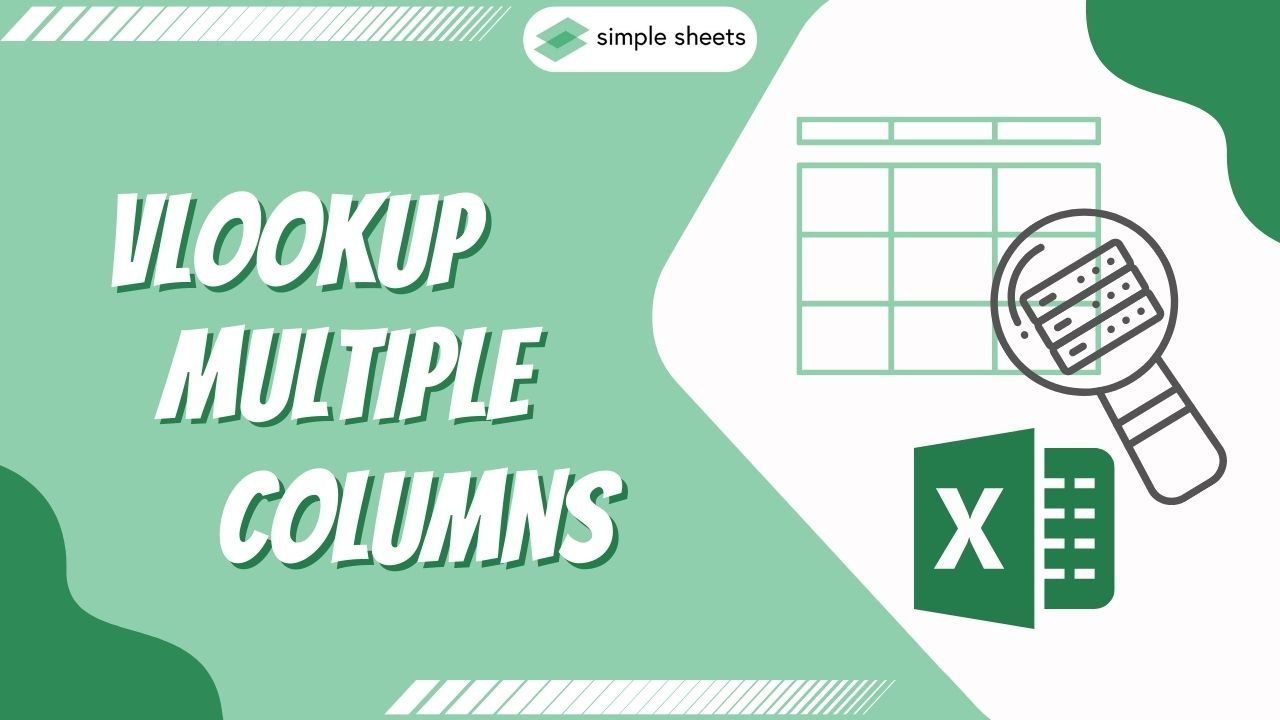Have you ever struggled with managing spreadsheet data and wished there was an easier way to look up values?

If you answered "yes" to that question, you should learn how to use the VLOOKUP function. It is an invaluable tool for organizing data quickly, and today, we will show you how to use it when dealing with multiple columns.

Not only can this save you time while setting up new worksheets, but it will also help keep your files neat, tidy, and efficient.

Read on as we cover the following:

• What Is VLOOKUP's Purpose?

• Excel VLOOKUP Function with Multiple Columns Syntax

• Return Exact Multiple Values

• Final Thoughts on VLOOKUP Multiple Columns

• Frequently Asked Questions on VLOOKUP Multiple Columns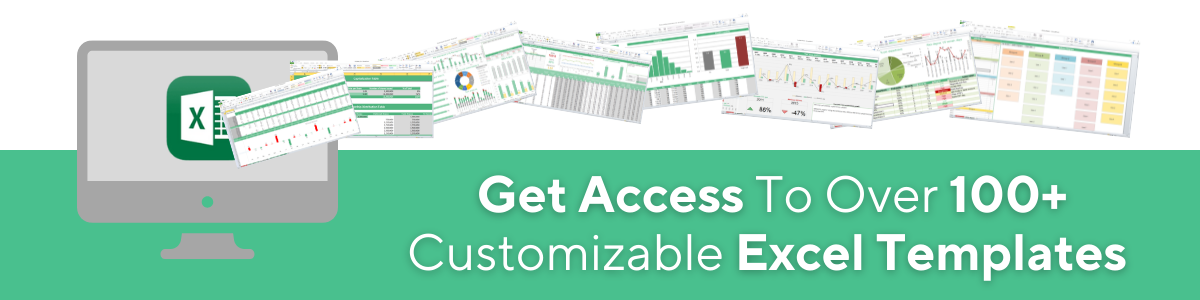## What Is VLOOKUP's Purpose?

The main purpose of VLOOKUP is to search for a value in the initial column of the table and then retrieve the corresponding value from another column on its right.

## Excel VLOOKUP Function with Multiple Columns Syntax

This function looks for a specific value in the first column of a table. It then adds up all the values in the same row but in columns to the right of the search column.

Excel VLOOKUP Multiple Columns Formula Breakdown: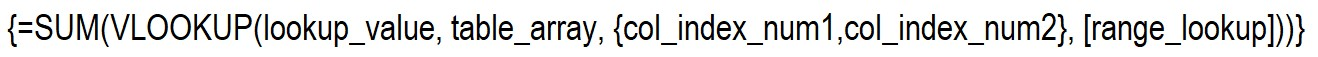What the formula means: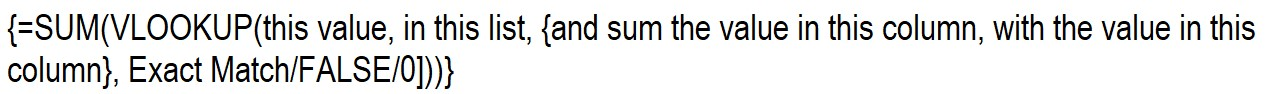## Return Exact Multiple Values

VLOOKUP has a limitation where it can only retrieve values from a single column, which is a disadvantage.

We must search for a match using the Item Description and Price in this example. You can't use the basic VLOOKUP syntax to return multiple values.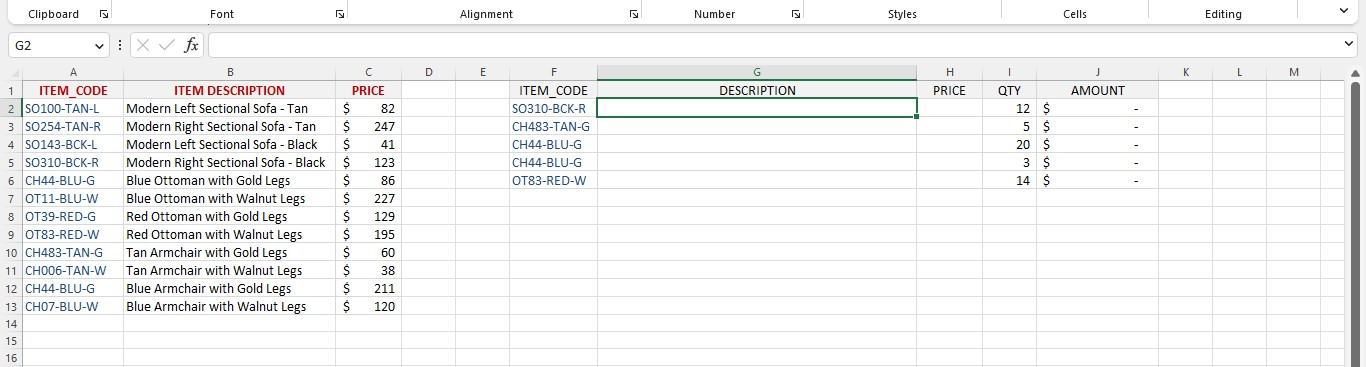To extract the description and price by matching the item code, you can modify the VLOOKUP formula using an array formula.

1. Select the first cell to put values in the columns Description and Price.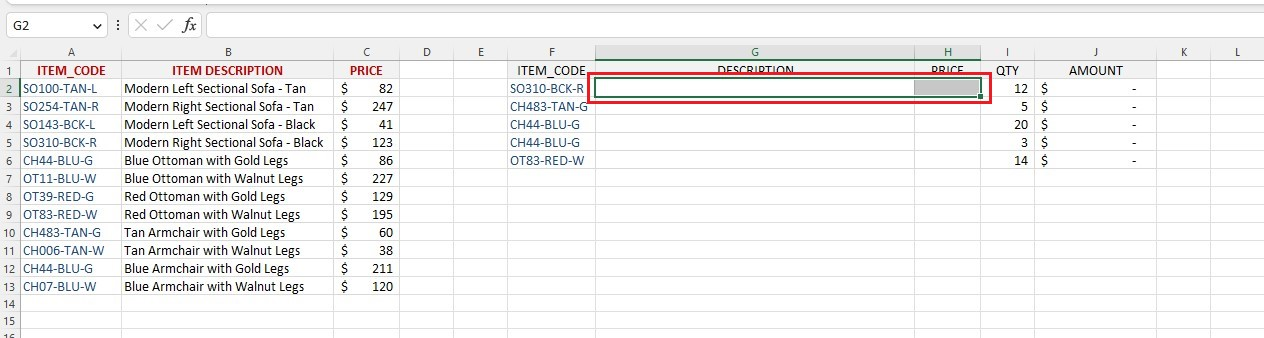1. Type the VLOOKUP function in the selected cell or the formula bar.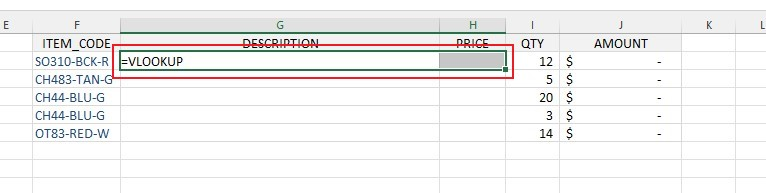1. Type the first argument, which is the LOOKUP value.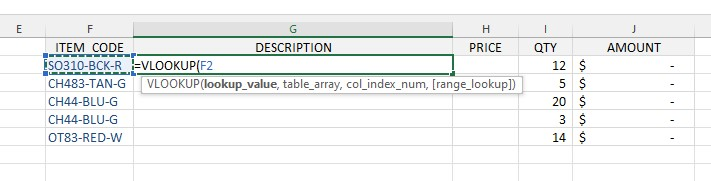1. Type the second argument, which is the Table Array.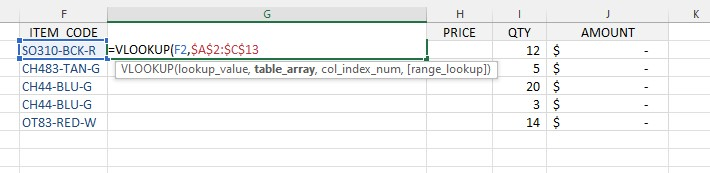Note: To retrieve the descriptions and prices for various item codes, select the Inventory table and remember to freeze the range by pressing F4.

1. Type the third argument.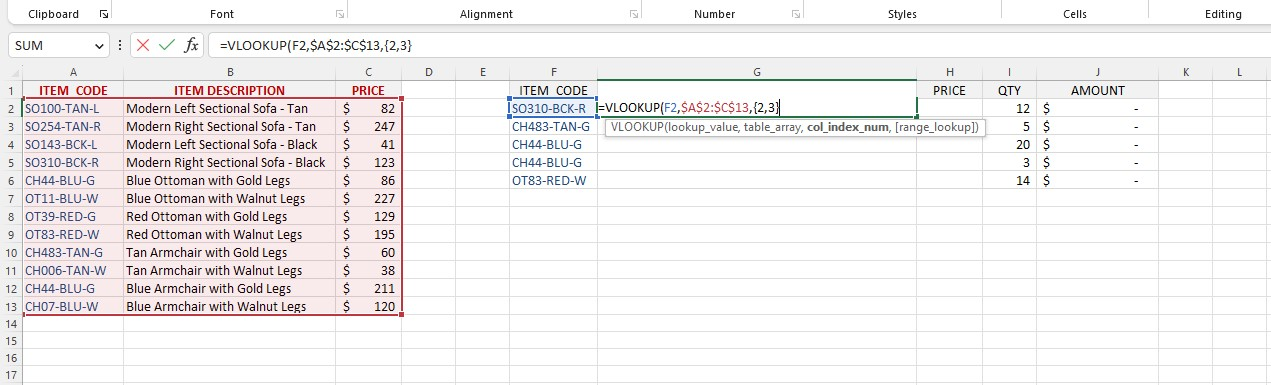Note: Retrieve columns 2 and 3 containing the description and price information.

1. Type the fourth argument, which is the range LOOKUP value.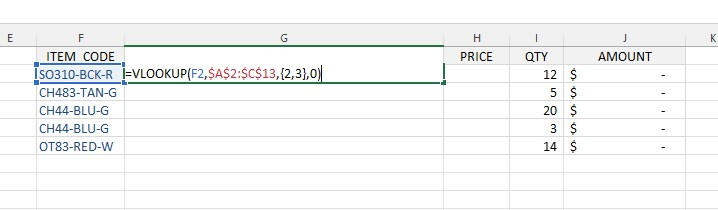Note: To ensure an exact match for the Item code, set this value to FALSE or 0.

1. To change the formula into an array function, press Ctrl + Shift + Enter.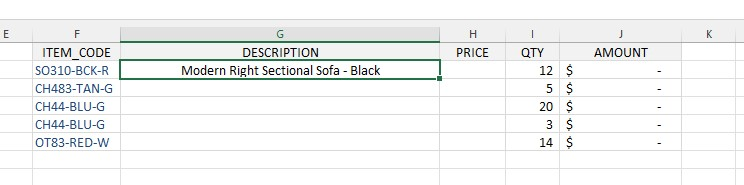Following the steps above, the formula will return multiple columns of the matching values based on multiple criteria in your Excel workbook.

## Final Thoughts on VLOOKUP Multiple Columns

The VLOOKUP formula is very helpful in Excel. It helps you find information quickly and correctly across different columns of data. Moreover, using our guide, you can use the VLOOKUP function more easily and quickly.

Visit Simple Sheets for more easy-to-follow guides, and remember to visit the related articles section of this blog post. For the most straightforward Excel video tutorials, subscribe to Simple Sheets on Youtube!## Frequently Asked Questions on VLOOKUP Multiple Columns

These are the frequently asked questions on VLOOKUP multiple columns in Excel:

### Can I use the VLOOKUP function for multiple sheets?

Yes. You can create a dynamic VLOOKUP INDIRECT formula to return the data from multiple sheets.

### Why does the SUM function with VLOOKUP return only one column value?

VLOOKUP looks in one column for a value and then finds the same one in another.

So, even though you're using SUM, it will only give you one answer because VLOOKUP can only give you one answer.

### Can I mix the CHOOSE and VLOOKUP functions for multiple criteria?

It is possible to combine the CHOOSE and VLOOKUP functions.

The CHOOSE function provides a value based on its index number, and the VLOOKUP function matches and returns the values.## Related Articles:

The Easiest Way to Connect a Slicer to Multiple Pivot Tables in Excel

Everything You Need To Learn About The Pivot Table In Excel

Everything You Need To Know About COUNT Formula In Excel

#### Want to Make Excel Work for You? Try out 5 Amazing Excel Templates & 5 Unique Lessons

We hate SPAM. We will never sell your information, for any reason.